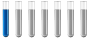## how do i balance this equation

Chemistry and homework help forum.

Organic Chemistry, Analytical Chemistry, Biochemistry, Physical Chemistry, Computational Chemistry, Theoretical Chemistry, High School Chemistry, Colledge Chemistry and University Chemistry Forum.

Share your chemistry ideas, discuss chemical problems, ask for help with scientific chemistry questions, inspire others by your chemistry vision!

Please feel free to start a scientific chemistry discussion here!

Discuss chemistry homework problems with experts!

Ask for help with chemical questions and help others with your chemistry knowledge!

Moderators: Xen, expert, ChenBeier

glnmgpnty
NewbiePosts: 1
Joined: Thu Sep 22, 2022 9:37 am

### how do i balance this equation

2AL(s) + 2KOH(aq) + H2SO4(aq) + 3H2SO41(aq) + K(aq) + Al(aq) + 2SO4(aq) + 12H2O(l) = 3H2(g) + K2SO4(aq) + 2H2O(l) + 2Al(aq) + 3SO4(aq) + KAl(SO4)2 * 12H2O
ChenBeier
Distinguished MemberPosts: 1227
Joined: Wed Sep 27, 2017 7:25 am
Location: Berlin, Germany